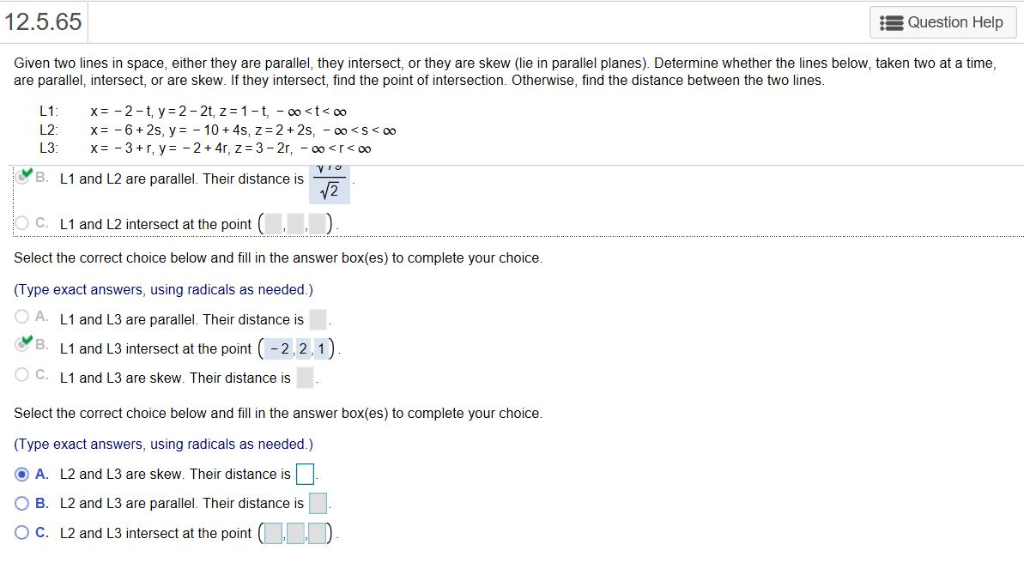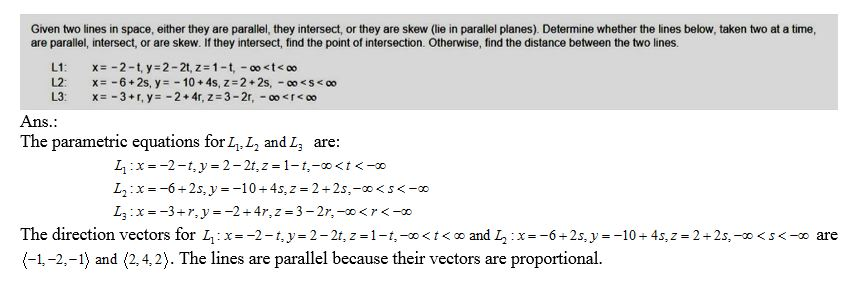# Given two lines in​ space, either they are​ parallel, they​ intersect, or they are skew​ (lie in parallel​ planes). Dete...

Given two lines in​ space, either they are​ parallel, they​ intersect, or they are skew​ (lie in parallel​ planes). Determine whether the lines​ below, taken two at a​ time, are​ parallel, intersect, or are skew. If they​ intersect, find the point of intersection.​ Otherwise, find the distance between the two lines.12.5.65 Question Help Given two lines in space, either they are parallel, they intersect, or they are skew (lie in parallel planes). Determine whether the lines below, taken two at a time are parallel, intersect, or are skew. If they intersect, find the point of intersection. Otherwise, find the distance between the two lines B. L1 and L2 are parallel. Their distance is C. L1 and L2 intersect at the point Select the correct choice below and fill in the answer box(es) to complete your choice (Type exact answers, using radicals as needed.) 0 A. L1 and L3 are parallel Their distance is B. L1 and L3 intersect at the point (-2.2.1) O C. L1 and L3 are skew. Their distance is Select the correct choice below and fill in the answer box(es) to complete your choice (Type exact answers, using radicals as needed.) @a. L2 and L3 are skew. Ther distance is □ O B. L2 and L3 are parallel. Their distance is ° C. L2 and L3 intersect at the point##### Add Answer of: Given two lines in​ space, either they are​ parallel, they​ intersect, or they are skew​ (lie in parallel​ planes). Dete...
More Homework Help Questions Additional questions in this topic.